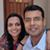3

Take an Angle T and the two sides S1 and S2

Draw a straight line on paper. Now draw another line that makes an angle T with it using your protractor. Call the point of intersection between the two lines A. Adjust your compass to length S1, and draw an arc with centre A intersecting your second line. Call the point where it intersects the line B. Now, adjust your compass to length S2, and draw a circle with centre at B. You will notice that it intersects the first line at two points, call them C and D.

Now, both triangles ABC and ABD meet your original specification (T, S1, S2). However, they are clearly not congruent.

For a visual demonstration of this idea, please see the following picture:

http://www.mathwarehouse.com/animated-gifs/images/why-ssa-does-NOT-prove-triangles-congruent-animation.gif

You can see their explanation at (using different, but equivalent language):

http://www.mathwarehouse.com/geometry/congruent_triangles/angle-side-side-postulate.phpVivekanand Vellanki
0

The important word in the SAS (side-angle-side) theorem is that the angle is the one subtended between the two sides - this is the angle between the two sides that are equal.